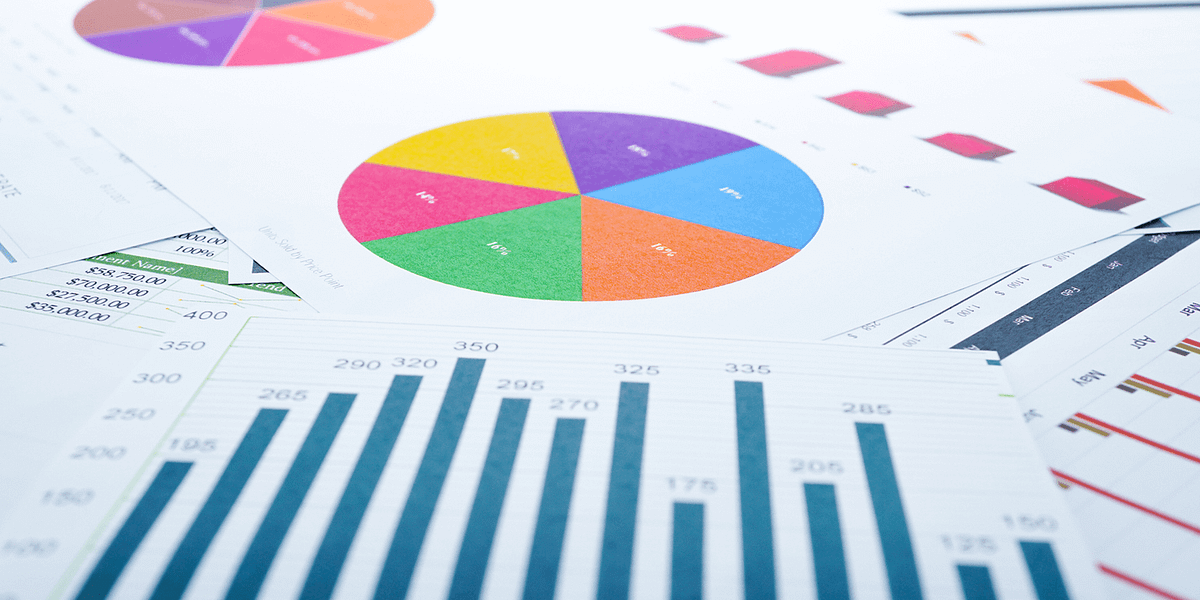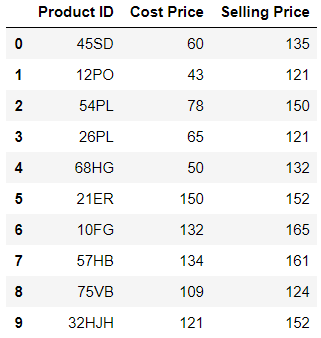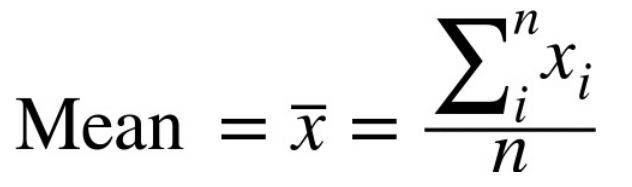# Descriptive Statistics with PandasStatistical concepts with examples, formula, and python code. The describe() function computes a summary of statistics pertaining to the DataFrame columns. This function gives the mean, std and IQR values. And, function excludes the character columns and given summary about numeric columns.

## Contents

1. Estimate of Location
• Mean
• Trimmed Mean
• Weighted Mean
• Median
• Mode

2.** Estimate of Variability**

• Deviation
• Mean Absolute Deviation
• Median Absolute Deviation
• Variance
• Standard Deviation
• Interquartile Range

3.** Correlation**

## Understanding the dataset

We will be using simple product details dataset which contains Product ID, Cost Price, and Selling Price to demonstrate various statistical methods.Most of the statistical methods can be done with Pandas except trimmed mean(scipy) and weighted mean(numpy). Reading product data into a data frame called ‘_products_’. Seaborn is a graphical plotting library.

``````import numpy as np
import pandas as pd
from scipy import stats
import seaborn as sns

## Estimate of Location

When there are several distinct values it is often very helpful to see an estimate of where the data is located or centered. It is also referred to as Measure of Central Tendency. Let’s see different ways of measurement.

1. Mean

The most basic estimate of location is the mean of data, simply an average of the values. That is the sum of all the values divided by the total number of values.

Example

Values: 10, 11 , 1, 20, 13

Mean = (10+11+1+20+13)/5 = 11The mean is symbolized as ‘x-bar’, n is the total number of values.

``````products['Cost Price'].mean()

Output: 94.2``````

## Master Pandas’ Groupby for Efficient Data Summarizing And Analysis

Learn to group the data and summarize in several different ways, to use aggregate functions, data transformation, filter, map.

## Exploratory Data Analysis is a significant part of Data Science

Data science is omnipresent to advanced statistical and machine learning methods. For whatever length of time that there is data to analyse, the need to investigate is obvious.

## 50 Data Science Jobs That Opened Just Last Week

Data Science and Analytics market evolves to adapt to the constantly changing economic and business environments. Our latest survey report suggests that as the overall Data Science and Analytics market evolves to adapt to the constantly changing economic and business environments, data scientists and AI practitioners should be aware of the skills and tools that the broader community is working on. A good grip in these skills will further help data science enthusiasts to get the best jobs that various industries in their data science functions are offering.

## Learn Data Science using CRISP-DM Framework

If you’re interested in the exciting world of data science, but don’t know where to start, CRISP-DM Framework is here to help.

## Statistical Tests for Data Analysis Part-I

These statistical tests allow researchers to make inferences because they can show whether an observed pattern is due to intervention or chance. There is a wide range of statistical tests.